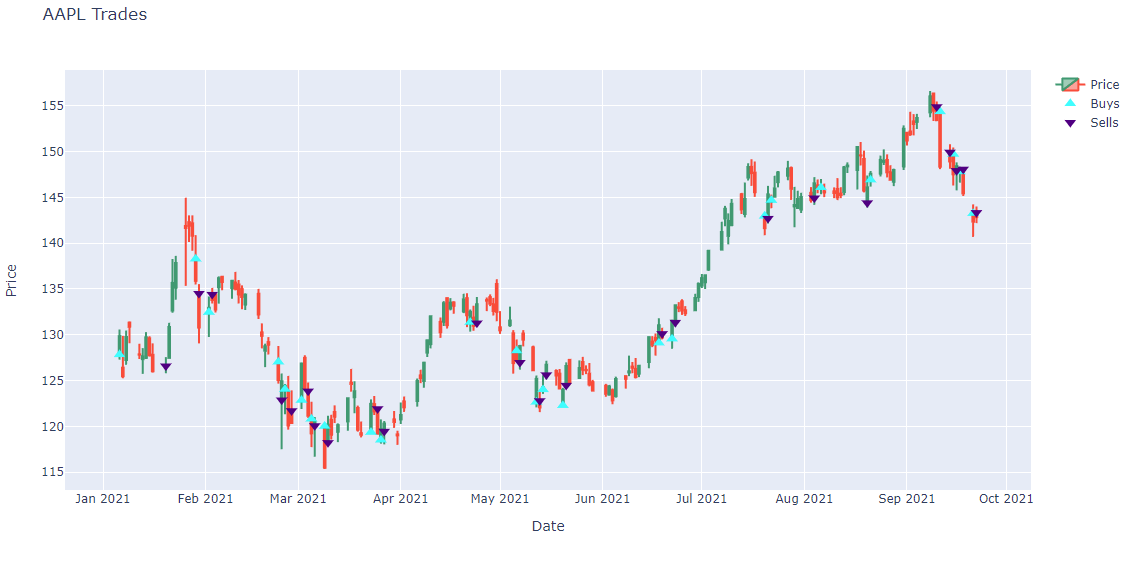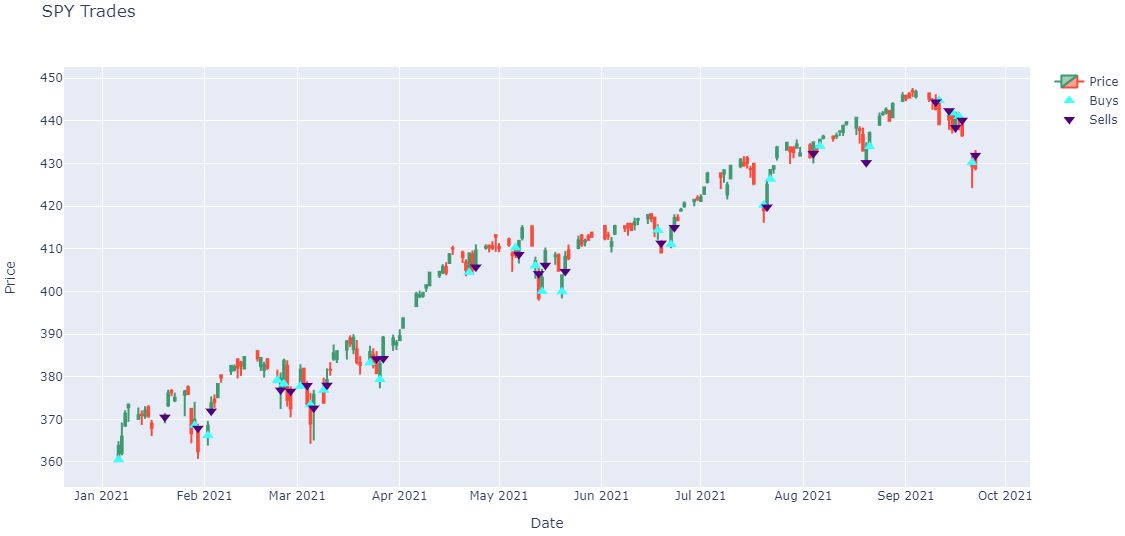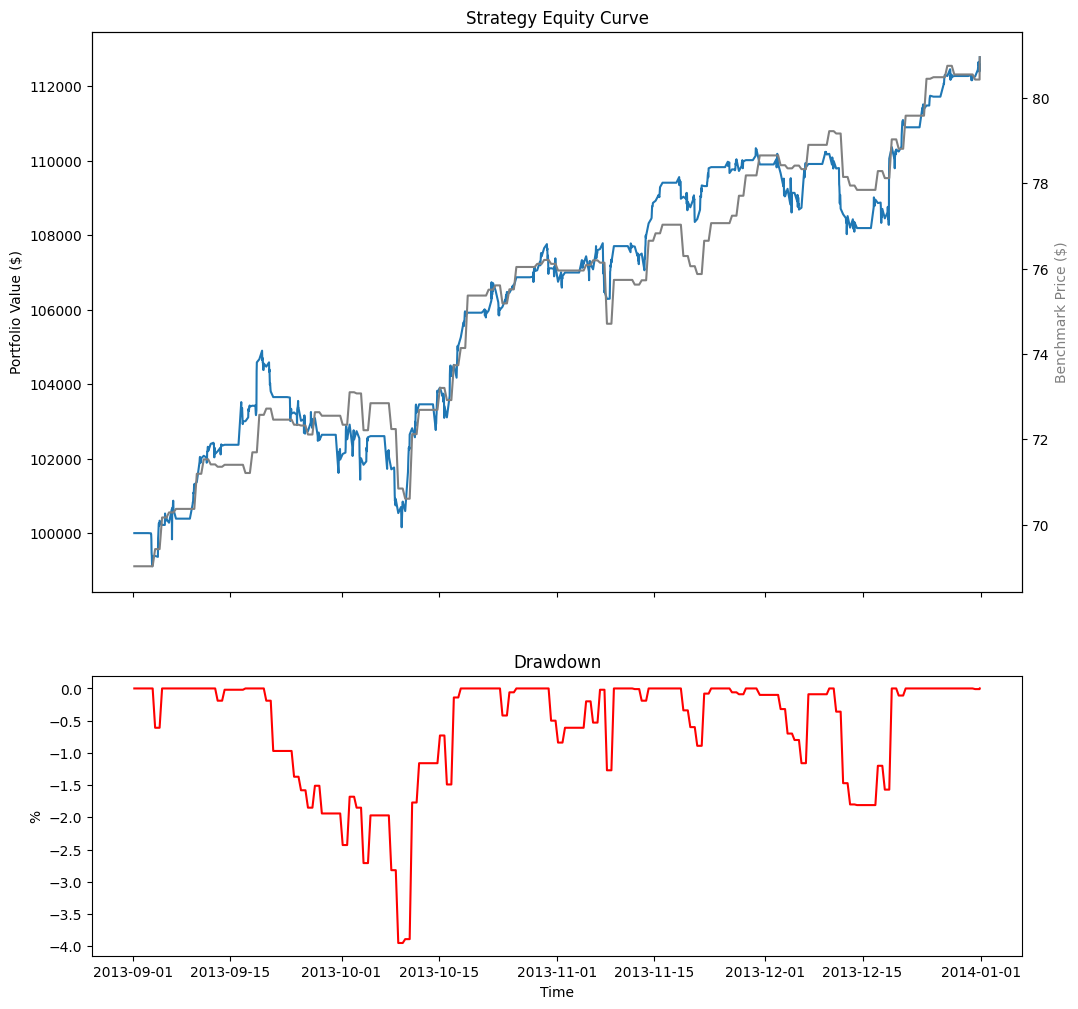# Meta Analysis

## Backtest Analysis

### Introduction

Load your backtest results into the Research Environment to analyze trades and easily compare them against the raw backtesting data. Compare backtests from different projects to find uncorrelated strategies to combine for better performance.

Loading your backtest trades allows you to plot fills against detailed data, or locate the source of profits. Similarly you can search for periods of high churn to reduce turnover and trading fees.

To get the results of a backtest, call the ReadBacktest method with the project Id and backtest ID.

#load "../Initialize.csx"

using QuantConnect;
using QuantConnect.Api;

var backtest = api.ReadBacktest(projectId, backtestId);
backtest = api.ReadBacktest(project_id, backtest_id)

To get the project Id, open the project in the Algorithm Lab and check the URL. For example, the project Id of https://www.quantconnect.com/project/13946911 is 13946911.

To get the backtest Id, open a backtest result in the Algorithm Lab and check the last line of its log file. An example backtest Id is 97e7717f387cadd070e4b77015aacece.

Note that this method returns a snapshot of the backtest at the current moment. If the backtest is still executing, the result won't include all of the backtest data.

The ReadBacktest method returns a Backtest object, which have the following attributes:

### Plot Order Fills

Follow these steps to plot the daily order fills of a backtest:

1. Get the backtest orders.
2. orders = api.ReadBacktestOrders(project_id, backtest_id)

To get the project Id, open the project in the Algorithm Lab and check the URL. For example, the project Id of https://www.quantconnect.com/project/13946911 is 13946911.

To get the backtest Id, open a backtest result in the Algorithm Lab and check the last line of its log file. An example backtest Id is 97e7717f387cadd070e4b77015aacece.

The ReadBacktestOrders method returns a list of Order objects, which have the following properties:

3. Organize the trade times and prices for each security into a dictionary.
class OrderData:
def __init__(self):
self.sell_fill_times = []
self.sell_fill_prices = []

order_data_by_symbol = {}
for order in orders:
if order.Symbol not in order_data_by_symbol:
order_data_by_symbol[order.Symbol] = OrderData()
order_data = order_data_by_symbol[order.Symbol]
(order_data.buy_fill_prices if is_buy else order_data.sell_fill_prices).append(order.Price)
4. Get the price history of each security you traded.
qb = QuantBook()
start_date = datetime.max.date()
end_date = datetime.min.date()
for symbol, order_data in order_data_by_symbol.items():
start_date -= timedelta(days=1)
all_history = qb.History(list(order_data_by_symbol.keys()), start_date, end_date, Resolution.Daily)
5. Create a candlestick plot for each security and annotate each plot with buy and sell markers.
import plotly.express as px
import plotly.graph_objects as go

for symbol, order_data in order_data_by_symbol.items():
history = all_history.loc[symbol]

# Plot security price candlesticks
candlestick = go.Candlestick(x=history.index,
open=history['open'],
high=history['high'],
low=history['low'],
close=history['close'],
name='Price')
xaxis_title='Date',
yaxis_title='Price',
xaxis_rangeslider_visible=False,
height=600)
fig = go.Figure(data=[candlestick], layout=layout)

marker=go.scatter.Marker(color='aqua', symbol='triangle-up', size=10),
mode='markers',
))

# Plot sells
x=order_data.sell_fill_times,
y=order_data.sell_fill_prices,
marker=go.scatter.Marker(color='indigo', symbol='triangle-down', size=10),
mode='markers',
name='Sells',
))

fig.show()
6.Note: The preceding plots only show the last fill of each trade. If your trade has partial fills, the plots only display the last fill.

Follow these steps to plot the equity curve, benchmark, and drawdown of a backtest:

1. Get the backtest instance.
2. backtest = api.ReadBacktest(project_id, backtest_id)

To get the project Id, open the project in the Algorithm Lab and check the URL. For example, the project Id of https://www.quantconnect.com/project/13946911 is 13946911.

To get the backtest Id, open a backtest result in the Algorithm Lab and check the last line of its log file. An example backtest Id is 97e7717f387cadd070e4b77015aacece.

3. Get the "Strategy Equity", "Drawdown", and "Benchmark" Chart objects.
4. equity_chart = backtest.Charts["Strategy Equity"]
drawdown_chart = backtest.Charts["Drawdown"]
benchmark_chart = backtest.Charts["Benchmark"]
5. Get the "Equity", "Equity Drawdown", and "Benchmark" Series from the preceding charts.
6. equity = equity_chart.Series["Equity"].Values
drawdown = drawdown_chart.Series["Equity Drawdown"].Values
benchmark = benchmark_chart.Series["Benchmark"].Values
7. Create a pandas.DataFrame from the series values.
8. df = pd.DataFrame({
"Equity": pd.Series({value.Time: value.Close for value in equity}),
"Drawdown": pd.Series({value.Time: value.Y for value in drawdown}),
"Benchmark": pd.Series({value.Time: value.Y for value in benchmark})
}).ffill()
9. Plot the performance chart.
10. # Create subplots to plot series on same/different plots
fig, ax = plt.subplots(2, 1, figsize=(12, 12), sharex=True, gridspec_kw={'height_ratios': [2, 1]})

# Plot the equity curve
ax.plot(df.index, df["Equity"])
ax.set_title("Strategy Equity Curve")
ax.set_ylabel("Portfolio Value ($)") # Plot the benchmark on the same plot, scale by using another y-axis ax2 = ax.twinx() ax2.plot(df.index, df["Benchmark"], color="grey") ax2.set_ylabel("Benchmark Price ($)", color="grey")

# Plot the drawdown on another plot
ax.plot(df.index, df["Drawdown"], color="red")
ax.set_title("Drawdown")
ax.set_xlabel("Time")
ax.set_ylabel("%")The following table shows all the chart series you can plot:

ChartSeriesDescription
Strategy EquityEquityTime series of the equity curve
Daily PerformanceTime series of daily percentage change
CapacityStrategy CapacityTime series of strategy capacity snapshots
DrawdownEquity DrawdownTime series of equity peak-to-trough value
BenchmarkBenchmarkTime series of the benchmark closing price (SPY, by default)
ExposureSecurityType - Long RatioTime series of the overall ratio of SecurityType long positions of the whole portfolio if any SecurityType is ever in the universe
SecurityType - Short RatioTime series of the overall ratio of SecurityType short position of the whole portfolio if any SecurityType is ever in the universe
Custom ChartCustom SeriesTime series of a Series in a custom chart

You can also see our Videos. You can also get in touch with us via Discord.